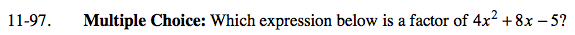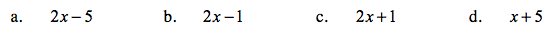Home > AC > Chapter 11 > Lesson 11.2.2 > Problem11-97

11-97.
1. Multiple Choice: Which expression below is a factor of 4x2 + 8x − 5? Homework Help ✎

1. 2x − 5

2. 2x − 1

3. 2x + 1

4. x + 5Factor the equation.

B. 2x − 1